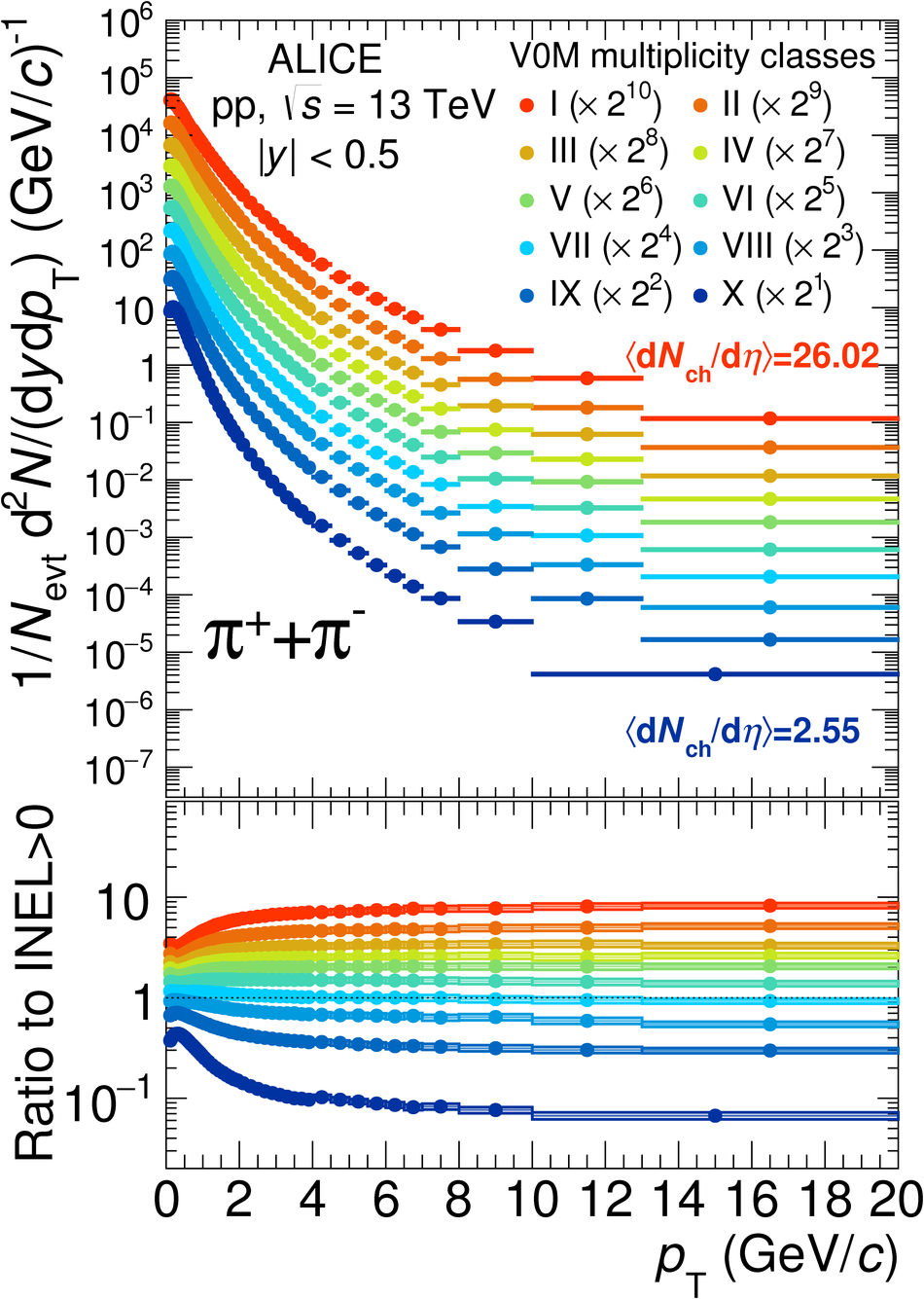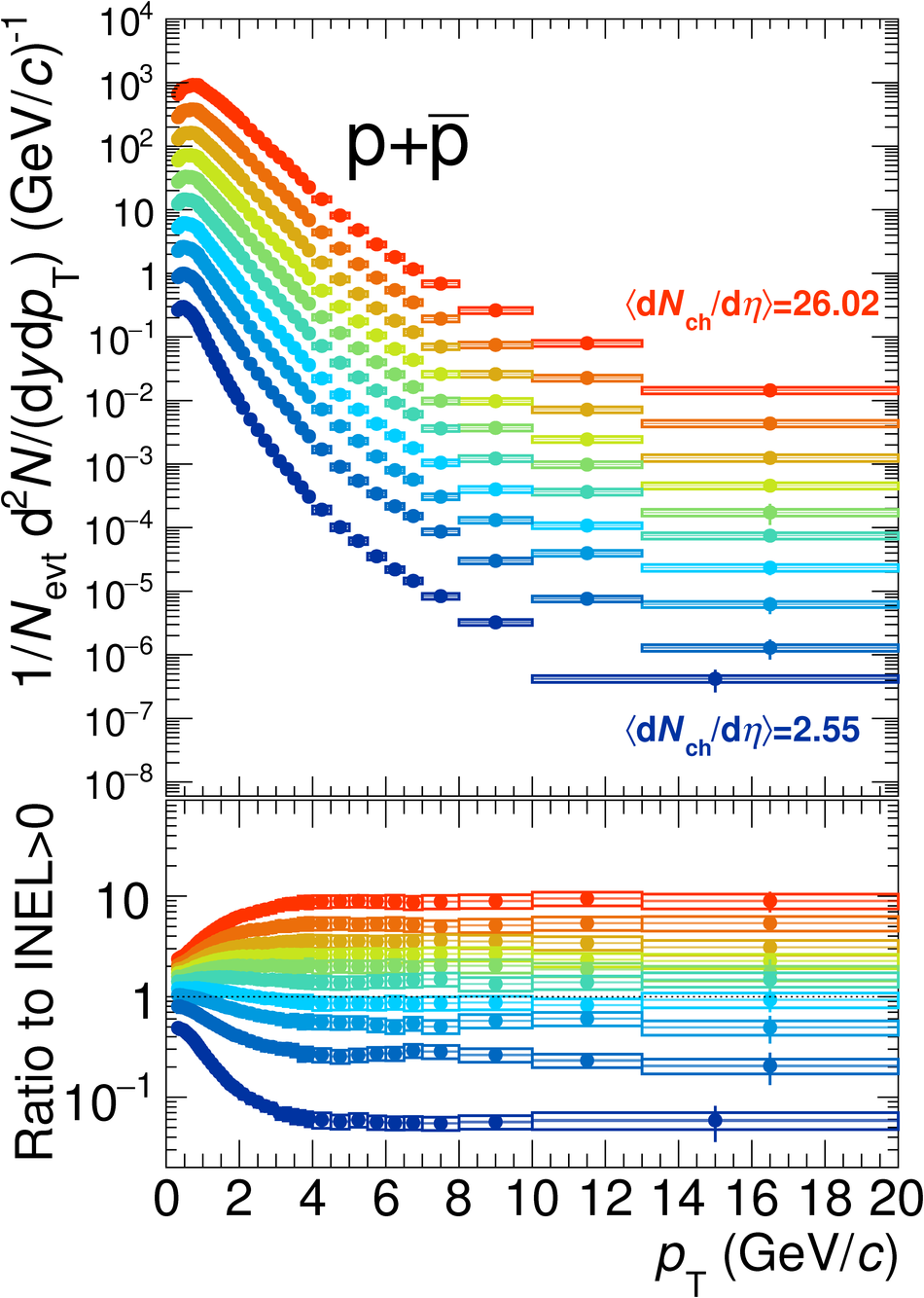# Multiplicity dependence of $π$, K, and p production in pp collisions at $\sqrt{s} = 13$ TeV

This paper presents the measurements of $\pi^{\pm}$, $\rm{K}^{\pm}$, $\rm{p}$ and $\bar{\rm{p}}$ transverse momentum ($p_{\rm{T}}$) spectra as a function of charged-particle multiplicity density in proton-proton (pp) collisions at $\sqrt{s}$ = 13 TeV with the ALICE detector at the LHC. Such study allows us to isolate the center-of-mass energy dependence of light-flavour particle production. The measurements reported here cover a $p_{\rm{T}}$ range from 0.1 GeV/$c$ to 20 GeV/$c$ and are done in the rapidity interval $|y|<~0.5$. The $p_{\rm{T}}$-differential particle ratios exhibit an evolution with multiplicity, similar to that observed in pp collisions at $\sqrt{s}$ = 7 TeV, which is qualitatively described by some of the hydrodynamical and pQCD-inspired models discussed in this paper. Furthermore, the $p_{\rm{T}}$-integrated hadron-to-pion yield ratios measured in pp collisions at two different center-of-mass energies are consistent when compared at similar multiplicities. This also extends to strange and multi-strange hadrons, suggesting that, at LHC energies, particle hadrochemistry scales with particle multiplicity the same way under different collision energies and colliding systems.

Submitted to: EPJC
e-Print: arXiv:2003.02394 | PDF | inSPIRE
CERN-EP-2020-024

Figures

## Figure 1

 Transverse momentum spectra of \pion, \kaon, and \pr for different multiplicity event classes. Spectra are scaled by powers of 2 in order to improve visibility. The corresponding ratios to INEL$>$0 spectra are shown in the bottom panels.